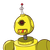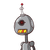# Rita bought 3 kg of rice at Rs 40 per kg and 2 kg of onion at Rs 20 per k. Howmuch total money she paid for the items.

Rita bought 3 kg of rice at Rs 40 per kg and 2 kg of onion at Rs 20 per k. How

much total money she paid for the items.​

### 2 thoughts on “Rita bought 3 kg of rice at Rs 40 per kg and 2 kg of onion at Rs 20 per k. How<br /><br />much total money she paid for the items.”

1.1 kg rice = ₹ 40

3 kg rice = ₹40×3 =₹120

1 kg onion = ₹20

2kg onion = ₹ 20× 2 =₹40

therefore, total money spent = ₹120+40=₹160.

2.Rice= 40x3kg
120 Rs

Onion=20x2kg
40 Rs

Total= rice + onion
= 120+40= 160 rs
Therefore, the total price rita paid is 160 rs